Latest Banking jobs   »

# Quantitative Aptitude Quiz For IDBI AM/Executive 2022- 3rd July

Directions (1-5): Table given below shows total number of votes cast in five cities. Total votes cast to X (in percentage) and total invalid votes cast. Only two people participate in the election (i.e., X and Y). Study the data carefully & answer the following questions.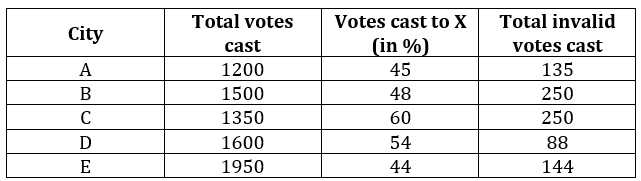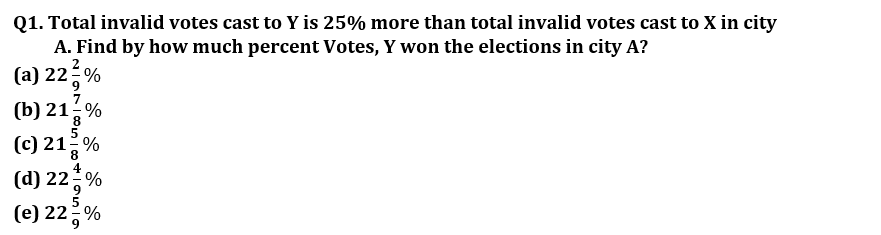Q2. Find the average number of total votes cast to Y in city A, C and E together?
(a) 736
(b) 748
(c) 764
(d) 754
(e) 760

Q3. Total invalid votes cast to Y in city D and city E is 25% and 50% respectively. Then total valid votes cast to Y in city D is what percent of total valid votes cast to Y in city E.
(a) 80%
(b) 75%
(c) 65%
(d) 60%
(e) 70%

Q4. Total votes cast to X in city C and D together is what percent of the total votes cast to Y in city A and B together?
(a) 110.25%
(b) 112.75%
(c) 114.25%
(d) 116.25%
(e) 118.25%

Q5. Total valid votes cast to Y in city B is how much more than total valid votes cast to Y in city C if total invalid votes cast to X in city B and City C is 84% and 92% respectively.
(a) 210
(b) 220
(c) 230
(d) 240
(e) 250

Directions (6-10): What approximate value should come in place of question mark (?)in the following questions?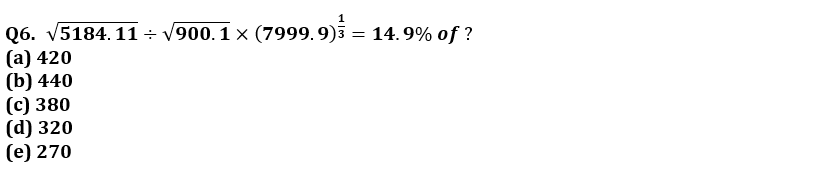Q7. 3? = 729.12 ÷ 81.11 × 242.91 ÷ 27.17 × 9.09
(a) 3
(b) 4
(c) 5
(d) 6
(e) 7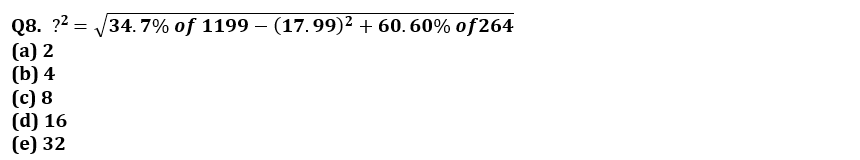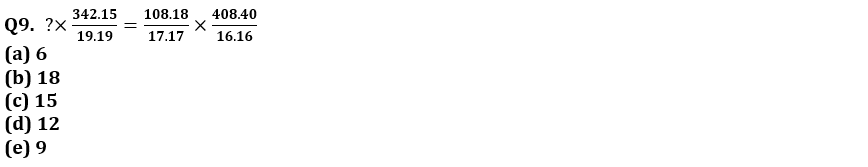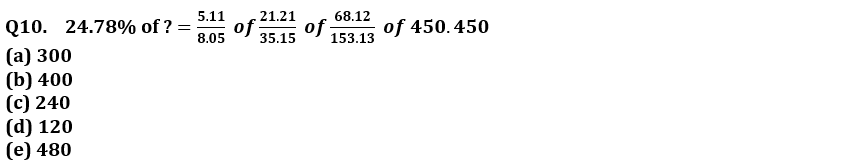Directions (11-15): The following questions are accompanied by two statements A and B. You have to determine which statements(s) is/are sufficient/necessary to answer the questions.

(a) Statement A alone is sufficient to answer the question but statement B alone is not sufficient to answer the questions.
(b) Statement B alone is sufficient to answer the question but statement A alone is not sufficient to answer the question.
(c) Both the statements taken together are necessary to answer the questions, but neither of the statements alone is sufficient to answer the question.
(d) Either statement A or statement B by itself is sufficient to answer the question.
(e) Statements A and B taken together are not sufficient to answer the question.

Q11. Find the overall profit or loss percentage of retailer if he sells two bats and four balls.
(A) Cost price of 3 bats is equal to selling price of 4 balls while profit percentage on selling one bat is 50%.
(B) On selling two bats retailer earn 50% profit while on selling four balls retailers loss 40% of his money.

Q12. A box contains 4 green ball, ‘x’ blue balls, 5 violet balls and ‘y’ yellow balls. Find the value of ‘x + y’.
(A) Probability of choosing two green ball is 1/20.
(B) Probability of choosing one blue ball or one violet ball is ½.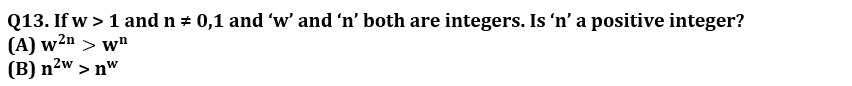Q14. Find the number of persons in a group if average weight of the group is 16?
(A) If one person left the group having weight 16 then average weight of the group is same as before.
(B) If two persons join the group having weight 16 and 28, then the average weight of group increases by 2.

Q15. In how many days ‘A’, ‘B’ and ‘C’ together can complete the work?
(A) A is 50% more efficient then B who is 100% more efficient than C while A and B together can complete the work in 12 days.
(B) ‘A’ and ‘B’, ‘B’ and ‘C’ and ‘C’ and ‘A’ together can complete the work in 12, 20 and 15 days respectively.

Solutions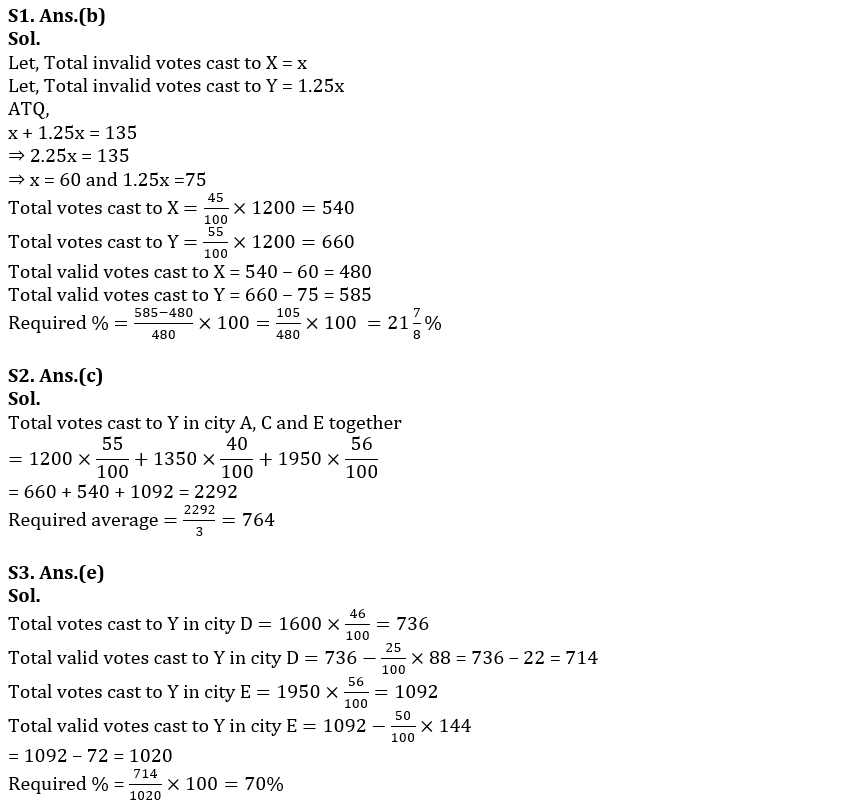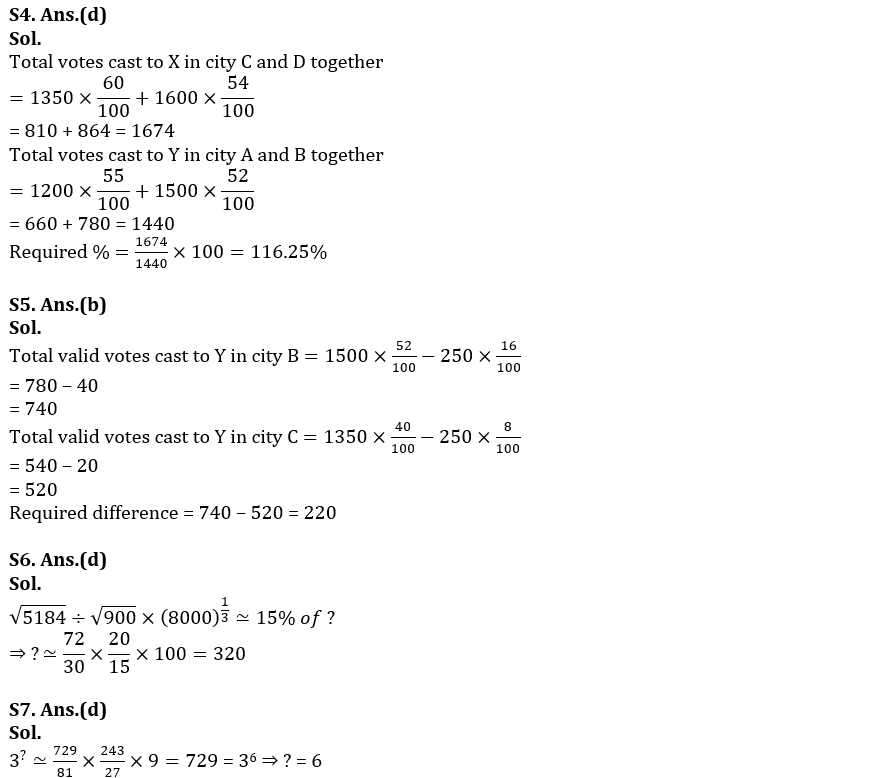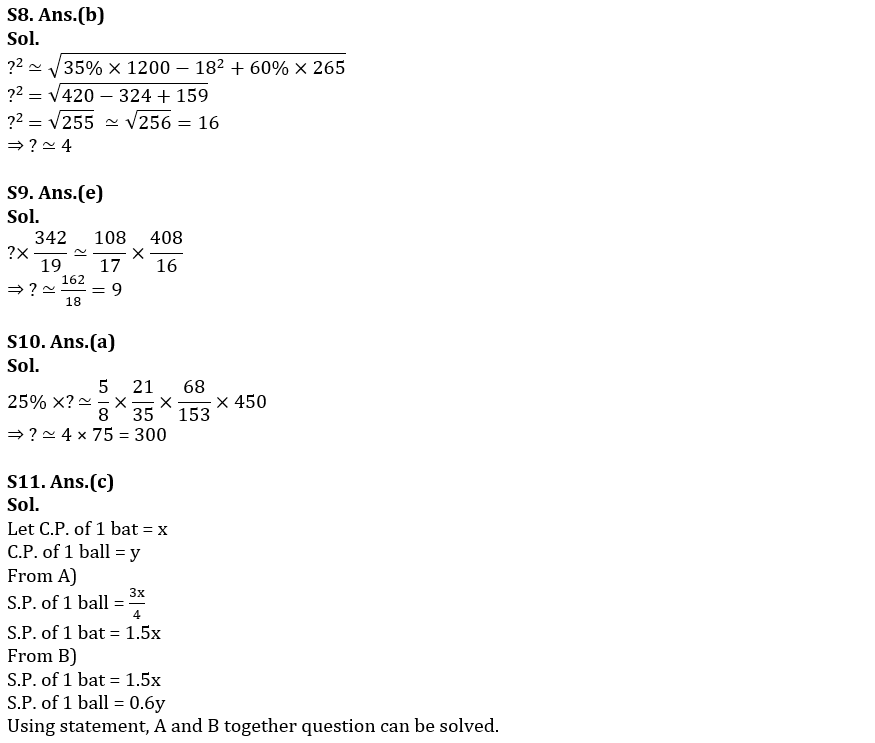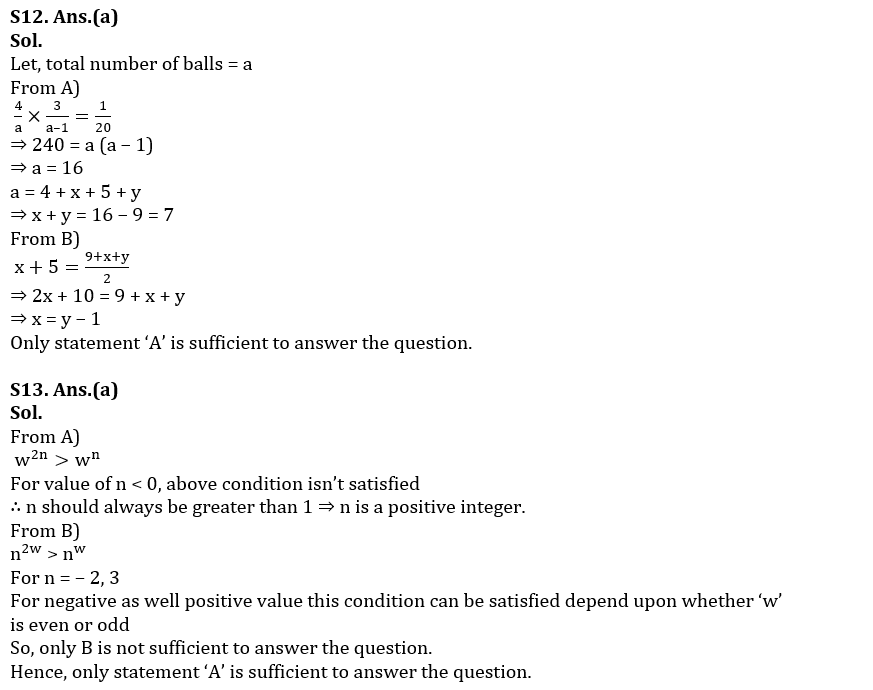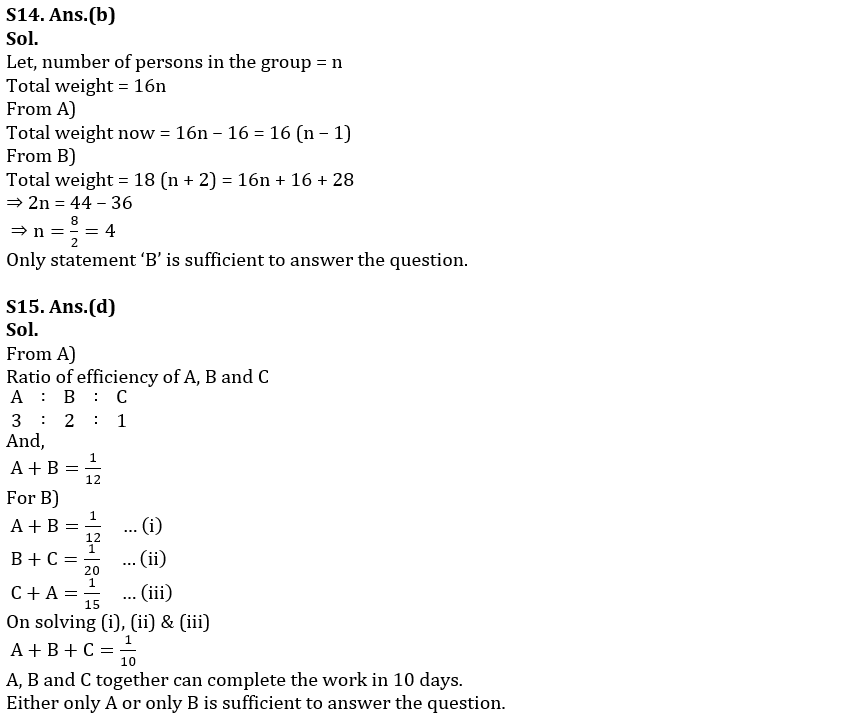#### Congratulations!Incorrect details? Fill the form again here

•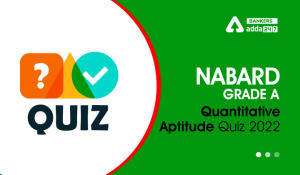Quantitative Aptitude Quiz For NABARD Gr...
•Quantitative Aptitude Quiz For IBPS RRB ...
•Quantitative Aptitude Quiz For NABARD Gr...
•Quantitative Aptitude Quiz For IBPS RRB ...
•Quantitative Aptitude Quiz For NABARD Gr...
•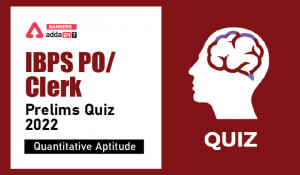Quantitative Aptitude Quiz For IBPS Cler...